M408D First Midterm, October 3, 2002

1. A sequence {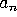} is said to ``grow faster'' than {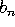} if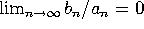. Put the following sequences in order of growth rate, from fastest to slowest. JUSTIFY YOUR ANSWERS.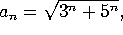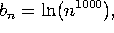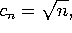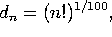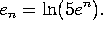2. Evaluate the following limits or improper integrals, or write DNE if the limits do not exist.

a)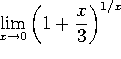b)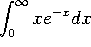c)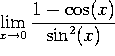d)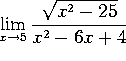3. Which of the following series converge, and which diverge. In each case, give a 1-sentence explanation (e.g., ``converges by ratio test'', or ``diverges by comparison to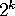'')

a)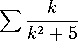b)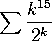c)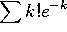d)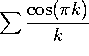4. a) Write down the Taylor series for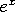.

b) Write down the 6-th order Taylor polynomial (i.e., through the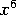term) for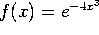.

c) Evaluate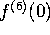. (That is, the 6-th derivative of f(x), evaluated at x=0.)

d) Evaluate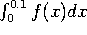to five decimal places. (No, you don't need a calculator!)

5. a) Find the second-order Taylor polynomial for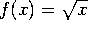around x=4.

b) What is the radius of convergence of the power series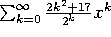? Justify your answer.